# Chapter 6: Trigonometry - Online Test

Q1. The value of sin2θ +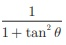is equal to
Explaination / Solution:
No Explaination.

Q2. tanθ cosec2θ - tan θ is equal to
Explaination / Solution: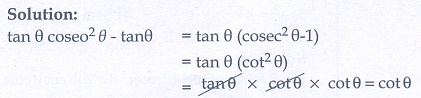Q3. If (sin a + coseca)2 +(cos a + sec a)2  = k + tan2a + cot2a , then the value of k is equal to
Explaination / Solution:Q4. If sin θ + cos θ = a and sec θ + cosecθ = b , then the value of b(a 2 -1) is equal to
Explaination / Solution:Q5. If 5x = sec θ and 5/x = tan θ, then x2 – (1/ x2) is equal to
Explaination / Solution: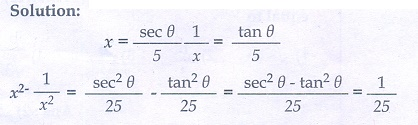Q6. If sin θ = cos θ , then 2 tan2θ + sin2θ −1 is equal to
Explaination / Solution: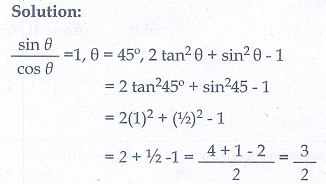Q7. If x = a tan θ and y = b sec θ then
Explaination / Solution: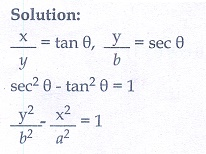Q8. (1 + tan θ + sec θ)(1 + cot θ − cosecθ) is equal to
Explaination / Solution:Q9. a cot θ + b cosecθ = p and b cot θ +a cosec θ = q then p 2 -q2  is equal to
Explaination / Solution: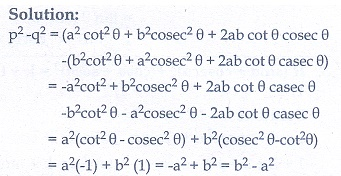Q10. If the ratio of the height of a tower and the length of its shadow is √3 : 1 then the angle of elevation of the sun has measure
Explaination / Solution: